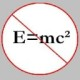You are currently browsing the tag archive for the ‘polynomial’ tag.

The following “polynomial-logarithmic” algebraic identity that one encounters on many occasions turns out to have a rather useful set of applications!

POLYNOMIAL-LOGARITHMIC IDENTITY: If$P(z)$ is a polynomial of degree$n \ge 1$ with roots$a_1, a_2, \ldots, a_n$, then$\displaystyle \frac{P'(z)}{P(z)} = \frac1{z-a_1} + \frac1{z-a_2} + \ldots + \frac1{z-a_n}$.

PROOF: This one is left as a simple exercise. (Hint: Logarithms!)

A nice application of the above identity is found in one of the exercises from the chapter titled Analysis (p120) in Proofs from the Book by Aigner, Ziegler and Hofmann.

EXERCISE: Let$p(x)$ be a non-constant polynomial with only real zeros. Show that$p'(x)^2 \ge p(x) p''(x)$ for all$x \in \mathbb{R}$.

SOLUTION: If$x = a_i$ is a zero of$p(x)$, then the right hand side of the above inequality equals zero, and we are done. So, suppose$x$ is not a root of$p(x)$. Then, differentiating the above identity w.r.t.$x$, we obtain$\displaystyle \frac{p''(x)p(x) - p'(x)^2}{p(x)^2} = - \sum_{k=1}^n \frac1{(x - a_k)^2} < 0$, and we are done.

It turns out that the above identity can also used to prove the well-known Gauss-Lucas theorem.

GAUSS-LUCAS: If$P$ is a non-constant polynomial, then the zeros of$P'$ lie in the convex hull of the roots of$P$.

PROOF: See this

HISTORY: The well-known Russian author V.V. Prasolov in his book Polynomials offers a brief and interesting historical background of the theorem, in which he points out that Gauss’ original proof (in 1836) of a variant of the theorem was motivated by physical concepts, and it was only in 1874 that F. Lucas, a French Engineer, formulated and proved the above theorem. (Note that the Gauss-Lucas theorem can also be thought of as some sort of a generalization (at least, in spirit!) of Rolle’s theorem.)

Even though I knew the aforesaid identity before, it was once again brought to my attention through a nice (and elementary) article, titled On an Algebraic Identity by Roberto Bosch Cabrera, available at Mathematical Reflections. In particular, Cabrera offers a simple solution, based on an application of the given identity, to the following problem (posed in the 2006 4th issue of Mathematical Reflections), the solution to which had either escaped regular problem solvers or required knowledge of some tedious (albeit elementary) technique.

PROBLEM: Evaluate the sum$\displaystyle \sum_{k=0}^{n-1} \frac1{1 + 8\sin^2 (k\pi /n)}$. (proposed by Dorin Andrica and Mihai Piticari.)

There is yet another problem which has a nice solution based again on our beloved identity!

PROBLEM: (Putnam A3/2005) Let$p(z)$ be a polynomial of degree$n$, all of whose zeros have absolute value 1 in the complex plane. Put$g(z) = p(z)/z^{n/2}$. Show that all zeros of$g'(z) = 0$ have absolute value 1.

In an earlier issue of Mathematical Reflections, Iurie Boreico (from Harvard) proposed the following problem.

Problem: A polynomial$p \in \mathbb{R}[X]$ is called a “mirror” if$|p(x)| = |p(-x)|$ . Let$f \in \mathbb{R}[X]$ and consider polynomials$p, q \in \mathbb{R}[X]$ such that$p(x) - p'(x) = f(x)$, and$q(x) + q'(x) = f(x)$. Prove that$p + q$ is a mirror iff$f$ is a mirror.

Two solutions – the pdf file size is around 1 Mb – to the above problem were proposed, and the one by the author is very close to the one I had worked out myself (partially) earlier but never really got around finishing it. So, I will post my solution here but at a slightly later time. In the meantime, you might be interested in finding a solution yourself.

### Blog Stats

• 346,159 hitsprof dr drd horia or… on My first postprof drd horia orasa… on My first postprof dr mircea orasa… on Inequality with lognotedscholar on Self-referential Paradoxes, In…prof dr mircea orasa… on Inequality with logprof dr mircea orasa… on Inequality with logprof dr mircea orasa… on 2010 in reviewkenji on Basic category theory, Iprof dr mircea orasa… on Solution to POW-10: Another ha…prof drd horia orasa… on Continued fraction for eprof drd horia orasa… on Inequality with logprof dr mircea orasa… on Solution to POW-12: A graph co…prof dr mircea orasa… on The Character of Physical…prof dr mircea orasa… on Milk and Tea puzzleprof drd horia orasa… on Inequality with log